# PSAT Math : How to graph a point

## Example Questions

### Example Question #1 : How To Graph A Point

A point at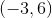in the standard coordinate plane is shifted right 5 units and down 3 units.  What are the new coordinates of the point?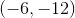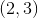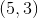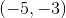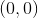Explanation:

The pointshifted to the right 5 units will shift along the x-axis, meaning that you will add 5 to the original x-coordinate, so the new. The point shifted down by three units will shift down the y-axis, meaning that you will subtract three from the original y-coordinate, so the new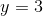.

The resultant coordinate is.

### Example Question #2 : How To Graph A Point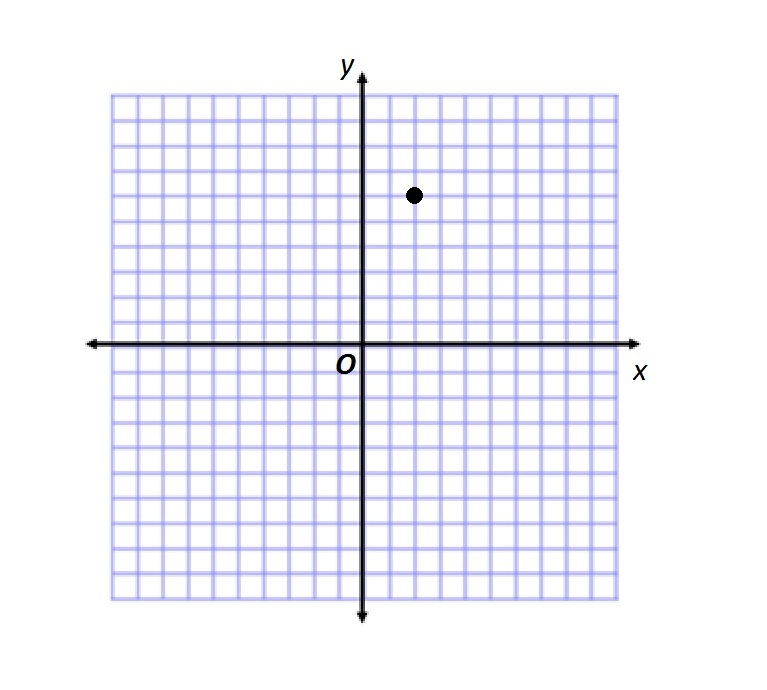Give the coordinates of the point plotted in the above set of coordinate axes.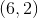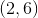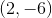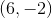None of the other responses is correct.